# Triangle numbers

## 0. Preliminary

Exercise

Write a function to find the triangle sum of any positive integer. The triangle sum of $n$ is the arithmetic sequence $1 + 2 + 3 + \dots + (n-1) + n$.

VBA skills:

• loops - the For...Next construct
• writing function procedures

What is meant by the "triangle sum"?

"A triangular number or triangle number counts objects arranged in an equilateral triangle" (Source: Wikipedia 2017)

Let $n$ represent the number of dots on one side of the triangle, then the triangle number $T_n$ has the formula $$\begin{equation}T_n=\sum_{k=1}^n = 1+2+3+\dots +(n-1)+n\end{equation}$$ see figure 1 were the dots on one side of the triangle can be envisaged as rows of dots of the same colour.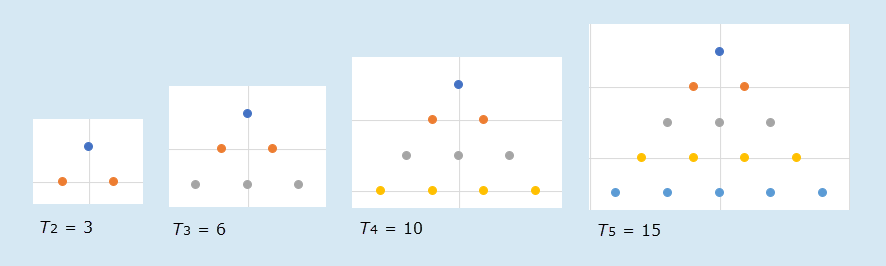Fig 1: Triangle numbers - $T_2$ has 2 rows with 3 dots, and $T_5$ has 5 rows with 15 dots

The sequence continues in figure 2.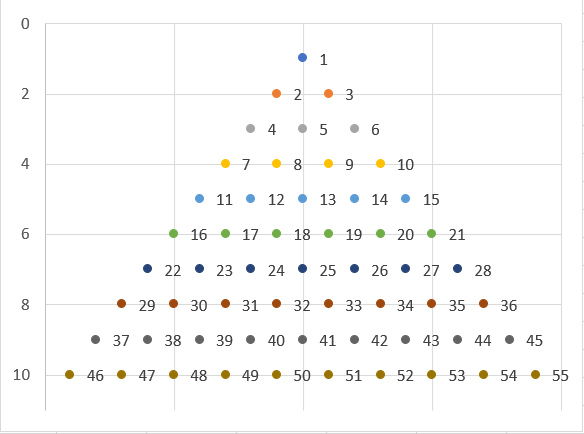Fig 2: Triangle numbers - n: Vertical (Value) Axis, and $T_n$ is the value on the right side of the triangle, for example $T_{10}=55$. The colours match figure 1

## 1. Suggested solution

Code 1: Macro Triangle write the data range for triangle n
Function Triangle(n As Long) As Long

'   This function calculates the triangular sum of a positive number

Dim x As Long, y As Long

y = 0
For x = 1 To n
y = y + x
Next x
Triangle = y

End Function


' Version 2 with error trapping for non positive n

Function Tri2(n As Integer) As Variant

Dim x As Long, y As Long

If n < 1 Then
Tri2 = CVErr(xlErrValue)
Else
y = 0
For x = 1 To n
y = y + x
Next x

Tri2 = y
End If

End Function



Code 1 - discussion:

• Question: What is the purpose of the code in Line 7: y = 0 ?.

• Answer: y = 0  is an initialization statement where the y variable is explicitly assigned a zero (0) value. The initial value of the variable is the starting value of the variable.

• The variable y is declared in line 5 as type Long. Although a Long data type has a value of zero by default, the initialization code emphasizes this point and explicit 0 is in line with the description of a "sequence of triangular numbers", namely 0, 1, 3, 6, 10, 15, … (sequence A000217 in the OEIS). The statement has no impact of the code's operation, and is included for readability

• Variable initialization of loop elements is demonstrated in Walkenbach (2007) p370

• Line 7 could be written as:
• ' Initialize
y = 0



## 2. Creating the triangle charts in Excel

A minimum of three data points $n => 2$ are required to create a triangle X Y chart.

### 2.1 Equilateral Triangle

The chart in figure 3 is an Excel X Y scatter chart with 10 rows. You can click the chart to view the data set.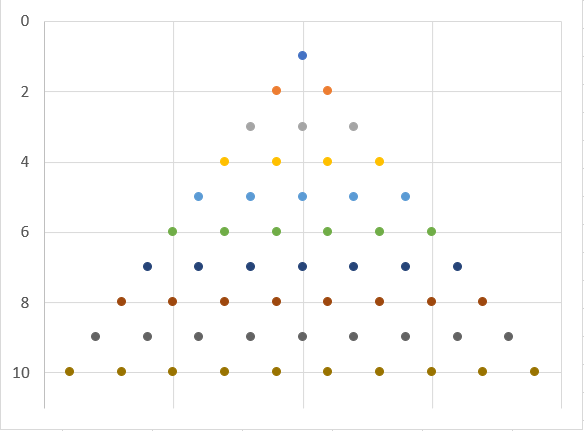Fig 3: Triangle chart x-y scatter plot with n = 10

### Create the data set

The data points for the chart in figure 1 were written to the worksheet with the TriangleData macro in code 2.

Code 2: Macro TriangleData write the data range for triangle n (min 2)
Sub TriangleData()

'     #
'    # #
'   # # #
'  # # # #

' interface connectors
Const n As Integer = 10
Const AnchorRef As String = "B4"

Dim x As Integer, y As Integer
Dim Anchor As Range
Dim Mid As Integer
Dim Max As Integer

Max = n * 2 - 1                     ' x axis 1 to Max (an odd number)
Set Anchor = Range(AnchorRef)

' write x vector
For x = 1 To Max
Anchor(x, 1).Value = x
Next x

Mid = (Max + 1) / 2             ' mid point of x vector

' write y vectors
For y = 0 To n - 1
For x = Mid - y To Mid + y Step 2
Anchor(x, y + 2).Value = y + 1
Next x
Next y

' select x-y data set (reverse order in chart axis)
Range(AnchorRef, Cells(x + 1, y + 2)).Select

End Sub


### Add the X Y (scatter) chart

To add the X Y chart:

• Select the data if not already selected by line 35 of code 2
• On the ribbon, select Insert > Charts > Scatter - the new chart will appear to be inverted with the point of the triangle at the base
• Right click the chart to display the (Chart Area) context menu and select Format Chart Area ... to display the Format Chart Area pane, then select Chart Options ▼ Vertical (Value) Axis item
• In the Axis Options section, tick Values in reverse order
• Adjust other format settings as appropriate

### 2.2 Lower Triangle

This version of the triangle is relevant to aspects of finance such as the portfolio variance-covariance matrix.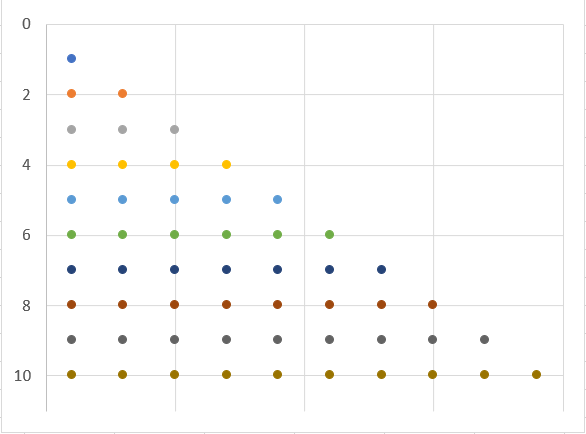Fig 4: Lower Triangle chart x-y scatter plot with n = 10

### Create the data set

The data points for the chart in figure 2 were written to the worksheet with the TriangleData2 macro in code 3.

Code 3: Macro TriangleData2 write the data range for triangle n (min 2)
Sub TriangleData2()

' #
' # #
' # # #
' # # # #

' interface connectors
Const n As Integer = 10
Const AnchorRef As String = "B24"

Dim x As Integer, y As Integer, z As Integer
Dim Anchor As Range
Dim Max As Integer

Max = n * 2 - 1                     ' x axis 1 to Max (an odd number)
Set Anchor = Range(AnchorRef)

' write x vector
For x = 1 To Max
Anchor(x, 1).Value = x
Next x

' write y vectors
For x = 1 To Max Step 2
z = z + 1
For y = z To n
Anchor(x, y + 1).Value = y
Next y
Next x

' select x-y data set
Range(AnchorRef, Cells(x + 1, y + 2)).Select

End Sub


To add the X Y chart, follow the steps in section 2.1.

## 3. Finance aspects

Equation 1 can be written as $n(n+1)/2$ as shown in equation 2 $$\begin{equation}T_n=\sum_{k=1}^n=1+2+3+\dots +(n-1)+n=\frac{n(n+1)}{2}=n(n+1)/2\end{equation}$$ For $n = 10$, $T_{10} = (10 \times 11)/2 = 55$

The lower triangle in figure 2 is equivalent to a lower triangle of a variance matrix. For a 10 asset portfolio there are 55 variance-covariance items. The number of portfolio covariance terms, items off the main diagonal is $T_n-n=55-10=45$ $$\begin{equation}T_n-n =\frac{n(n+1)}{2}-n=\frac{n^2+n}{2}-\frac{2n}{2}=\frac{n^2-2n+n}{2}=\frac{n(n-1)}{2}=n(n-1)/2\end{equation}$$ For $n = 10$, $T_{10}-n =(10 \times 11)/2-10=55-10=45$ covariance items (off diagonal) and $10$ variance items (on diagonal) - see figure 5.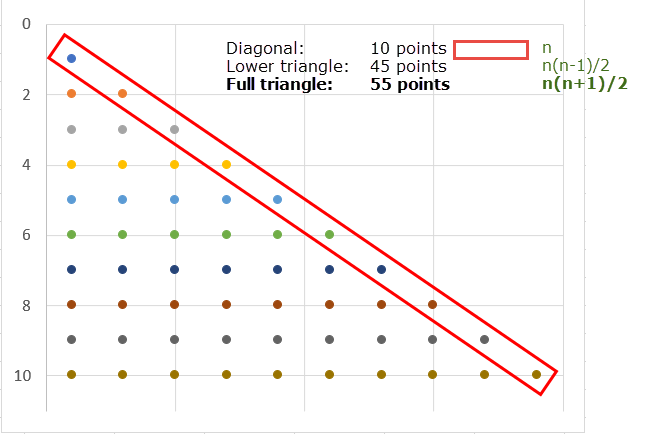Fig 5: Lower Triangle and Diagonal chart x-y scatter plot with $n = 10$

Other applications in finance include, Pascal's triangle, and binomial trees.

• References
• Download the Excel file for the images in this module: xlf-triangle.xlsx [36 KB]
• Development platform: Excel 2016 (64 bit) Office 365 ProPlus on Windows 10
• Published: 4 November 2017
• Revised: Saturday 25th of February 2023 - 10:12 AM, [Australian Eastern Time (AET)]